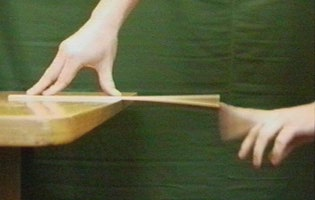How and why does the height of water in a glass affect it's resonance? (resonant frequency)

Why does the resonance frequency of a vibrating wine glass changes with the level of water in the wine glass?

The resonant frequency will be made lower the more water is in the glass, because the glass must vibrate against the water, increasing the effective inertia in the glass. Resonant frequency always looks like the square root of some kind of restoring force divided by some kind of inertia, so increasing inertia reduces resonant frequency. Or another way to look at it is, the resonant frequency is inversely proportional to the time it takes a wave to pass down the glass and bounce back up again, and contact with dense water slows down that wave.

Note this is the opposite effect that you get when you blow over the top of a wine bottle-- there the wave is in the air, not the glass, and the resonant frequency is inversely proportional to the time for sound waves in air to propagate down through the air and bounce back up again. That will be a very obvious effect, increasing the water level raises the resonant frequency in the air dramatically. But it doesn't sound like that is what you are talking about.

Note that the other answer raises the possibility that the vibration in the glass might actually bounce when it reaches the water level, much like the sound waves in the air, in which case raising the water level would raise the frequency. So your experiment will tell which of these effects is actually happening, because if the wave has to still go all the way to the bottom of the glass before coming back up, then raising the water level will lower the resonant frequency.

The parts of the glass that are in contact with the water, cannot oscillate freely, they have to displace the water next to them.

The parts of the glass above the water level can oscillate freely. The eigenfrequency of the glass thus depends on how much of the glass has to oscillate with water and how much without.

Place a long ruler halfway over the edge of a table, then hit it to make it oscillate like in the image below. (click the image to find its source, which is a related article)Changing how much percent of the ruler is on the table changes the frequency of the oscillation of the free end (which gets longer or shorter).

Your wine glass is similar. The part of the glass that's in contact with the water is similar to the part of the ruler that's on the table.

What is resonance?

Resonance is a phenomenon in which external vibrations (or forces) causes another system to oscillate at a particular frequency with large amplitude.

In your case, the resonance is caused by the speakers in the air column (the glass acts as an air column).

=> The speakers cause the air in the glass to vibrate at its resonance frequency

=> The air inside the glass is the affected system

Mathematical DerivationThe figure above shows sound waves vibrating inside an air column (glass in your case).

At the base of the air column, the air cannot vibrate; hence, there must be a displacement node at that point. At the top of the air column, the air cannot stay at one position as the air is moving at the top; therefore, the air at the top vibrates with the maximum amplitude.

Let the length of the air column be $L$.

The following formulae will be used in this derivation.

$$v = f\lambda, \space \space f = \frac{v}{\lambda}$$

where $v$ is the speed of sound in air, $f$ is the frequency of the vibrations, $\lambda$ is the wavelength of the vibrations.

Case 1:

The air column contains $\frac{\lambda}{4}$ of the wave.

$$L = \frac{\lambda}{4}, \space \space 4L = \lambda$$

$$f = \frac{v}{4L}$$

Case 2:

The air column contains $\frac{3\lambda}{4}$ of the wave.

$$L = \frac{3\lambda}{4}, \space \space \frac{4}{3}L = \lambda$$

$$f = \frac{3v}{4L}$$

Case 3:

The air column contains $\frac{5\lambda}{4}$ of the wave.

$$L = \frac{5\lambda}{4}, \space \space \frac{4}{5}L = \lambda$$

$$f = \frac{5v}{4L}$$

 You can observe a pattern. All the possible resonance frequencies can be summarized using the following forumla:

$$f = (2n + 1)\frac{v}{4L}$$

How does the level of water affect the experiment?

As you might have observed in the derivation, the resonance frequencies of the air column depend on the length of the air column. In your case, the base of the water (water height/water level) acts as the lower end of the air column. The air vibrates between the top of the air column and the base of the water height. Therefore, changing the water level effectively changes the air column's height which in turn changes the resonance frequencies.

The vibrating air column's oscillations are transferred to the wine glass. So understanding how the water level affects the frequency of the air in the column is as good as understanding how the water level affects the resonance frequency of the wine glass.